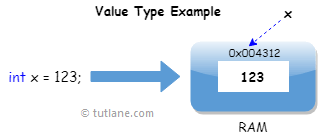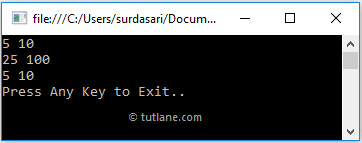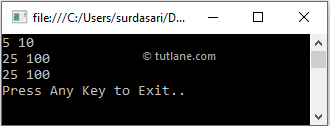# C# Value Type and Reference Type with Examples

In c#, we have two ways to allocate the space in memory, i.e., either on stack or heap memory based on the Value Type or Reference Type parameters.

In the previous chapter, we learned about Data Types in C# and how the Data Types in c# are categorized as a Value Type or Reference Type with examples.

We will now learn the value types and reference types in c# and how the Value Type and Reference Type parameters will hold the data and memory in the same location with examples.

## C# Value Types

In c#, a data type is a Value Type if it holds the value of the variable directly on its own memory space, and Value Types will use Stack memory to store the values of the variables.

For example, if we define and assign a value to the variable like int x = 123; then the system will use the same memory space of variable ‘x’ to store the value ‘123’.

Following is the pictorial representation of value types in the c# programming language.The following are the different data types that will fall under Value Type in c# programming language.

 int float long char bool byte decimal double enum sbyte short struct uint ulong ushort

## C# Pass Value Type by Value

In c#, if we pass a value type variable from one method to another method, the system will create a separate copy for the variable in another method. If we make changes to the variable in one method, it won’t affect the variable in another method.

Following is the example of passing the value type by value in c# programming language.

using System;

namespace Tutlane
{
class Program
{
static void Square(int a, int b)
{
a = a * a;
b = b * b;
Console.WriteLine(a + " " + b);
}
static void Main(string[] args)
{
int num1 = 5;
int num2 = 10;
Console.WriteLine(num1 + " " + num2);
Square(num1, num2);
Console.WriteLine(num1 + " " + num2);
Console.WriteLine("Press Enter Key to Exit..");
}
}
}

If you observe the above example, we defined two variables (num1, num2) in the Main() method, and we are making changes to those variables by passing them to the Square() method, but those changes won’t affect the variables in Main() method.

When we execute the above program, we will get the result as shown below.If you observe the above result, the changes made to variables in the Square() method didn’t affect the variables in the Main() method.

## C# Reference types

In c#, Reference Types will contain a pointer that points to another memory location that holds the data. The Reference Types won’t store the variable value directly in its memory. Instead, it will store the memory address of the variable value to indicate where the value is being stored.

For example, if we define and assign a value to the variable like string name = "Suresh Dasari"; then the system will store the variable value “Suresh Dasari” in one location and the variable "name" in another location along with the memory address of the variable value.

Following is the pictorial representation of reference type in c# programming language.The following are the different data types that will fall under Reference Type in c# programming language.

## Pass Reference Type by Value

In c#, if we pass a reference type variable from one method to another method, the system won’t create a separate copy for that variable. Instead, it passes the address of the variable, so if we make any changes to the variable in one method, that also reflects in another method.

Following is the example of passing the reference type by value in the c# programming language.

using System;

namespace CsharpExamples
{
class Person
{
public int age;
}
class Program
{
static void Square(Person a, Person b)
{
a.age = a.age * a.age;
b.age = b.age * b.age;
Console.WriteLine(a.age + " " + b.age);
}
static void Main(string[] args)
{
Person p1 = new Person();
Person p2 = new Person();
p1.age = 5;
p2.age = 10;
Console.WriteLine(p1.age + " " + p2.age);
Square(p1, p2);
Console.WriteLine(p1.age + " " + p2.age);
Console.WriteLine("Press Any Key to Exit..");
}
}
}

If you observe the above example, we created a new class called “Person” and created an instance of a new class (Person) and assigned values to the variables in Main() method, and we are making changes to those variable by passing it to Square() method and those changes will be reflected in Main() method.

When you execute the above program, you will get the result as shown below.If you observe the above result, the changes we made to variables in the Square() method also reflected for the variables in the Main() method.

This is how we can use Value Type and Reference Type in c# programming language based on our requirements.